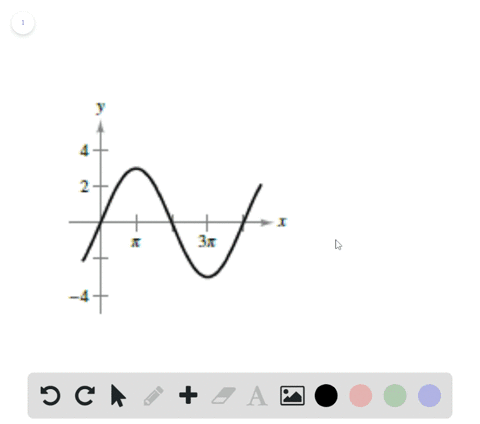🎉 The Study-to-Win Winning Ticket number has been announced! Go to your Tickets dashboard to see if you won! 🎉View Winning Ticket### Graphical Reasoning In Exercises 67 and $68,$ fin…

01:45University of Southern California
Problem 66

# Sketching the Graph of a Trigonometric Function In Exercises $55-66,$ sketch the graph of the function.$$y=1+\sin \left(x+\frac{\pi}{2}\right)$$

## Discussion

You must be signed in to discuss.

## Video Transcript

why of X plus pi divided by two. Now, when we graft this function, Um, one way that would make it very easy to graph it would be to look at the general form of sign. So I'm going to write out a generalized expression. It could represent any modification of sign Whitehall's D plus a sign of B X plus C. And with this, we can examine each of these constants I've tacked on to our original sign equation and see how they affect or transform our graph. Now, when we look over here, we see that r D equals one. So I'm gonna write that right here. D equals one, and that devalue is responsible for a vertical shift. So when we go to graph our function, we know that in comparison to the parent functions sine of X, it's going to be shifted up one value or yeah, one unit, all values of sign will be shifted up. One unit moving on to a A is in charge of our amplitude. And if we look over here a equals one. Okay, that means that the amplitude is gonna also be one looking next to be We see that B is also one b is responsible for a period and a period for any function of sign equals to pi divided by being. And since that's one and just equals two pi. So the period of our function is gonna be the same as the original sine function just to pie. And we see that our see value is positive. Pi house. Now, since C equals, perhaps it's responsible for the horizontal shift. And since it's a positive value in this case, all the values of our graph are going to shift towards the left in the negative direction since we're adding on the inside of our function. All right, so to make these transformations much more obvious, I'm gonna graft everything in comparison with the parent function. Why equal sign X. So we know that for sine X, it has a maximum value of pi house zeroes out. Hi. Minimum value of three by halfs. Right there comes back to zero it to pie. And that behavior repeats this way. All right, so our graph shows two periods of our function just trying to trace it as accurately as possible. Remember that it is a sketch, though So it's okay if it gets a little messy. That sign X and now in green gonna graph our function. All right, so we know that everything has been shifted up. One unit. Well, how about next? I'm gonna graft that. That shift in one in blue. So everything gets shifted up. One unit. Meaning, uh, excuse the sirens in the background, huh? All right, So here, Jonah blue graph. And this is why equals one plus sign X and then in green. I'm going to account for that horizontal shift, All right? And we'll see that everything moves to the left. So this value right here comes this way. Okay? Yeah, yeah. Just gotta think about it. Everything comes back by half, and then we'll see. That's the function of y equals one plus sign of X plus pi divided by two. And that green graph right there. Ease the graph of our function